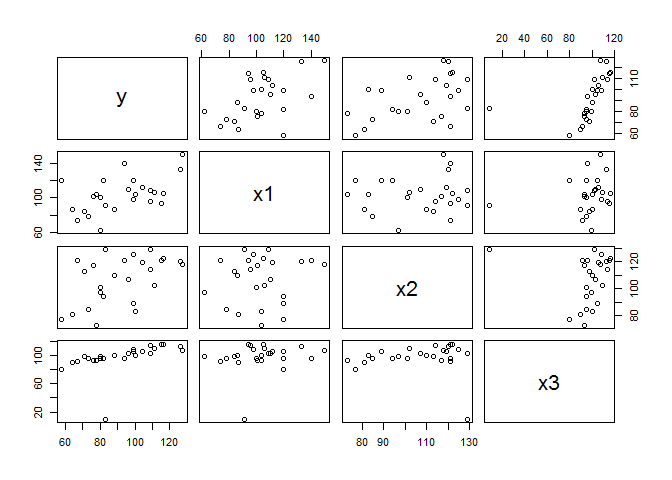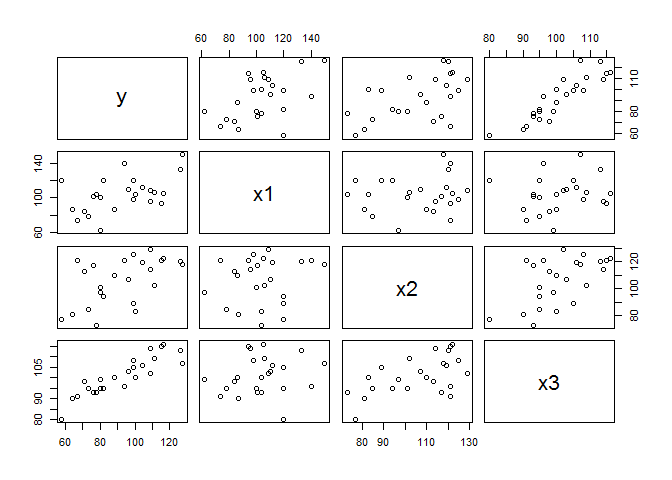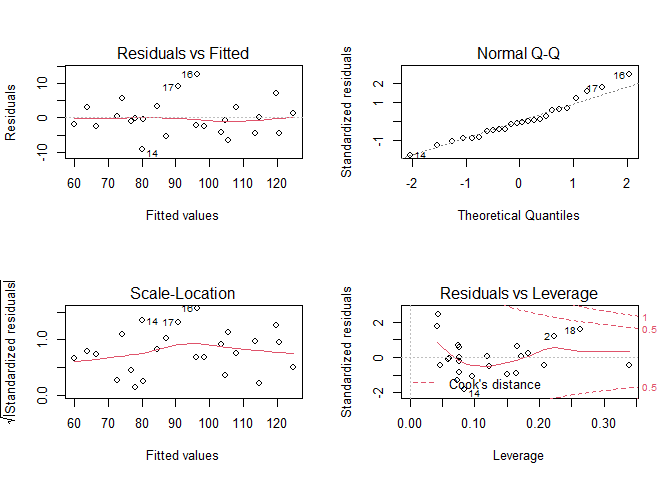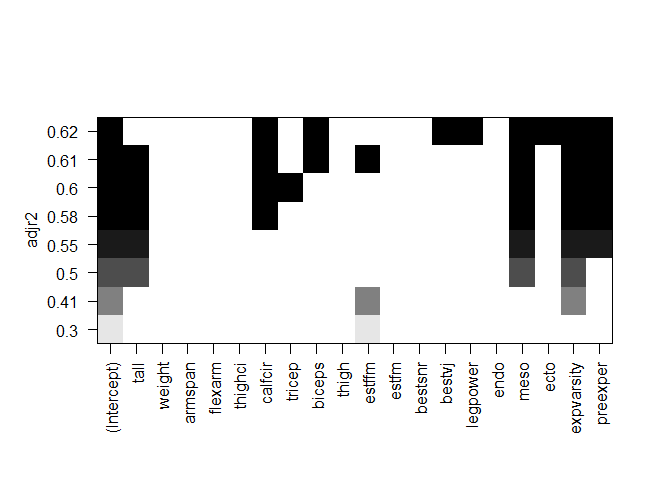# Multiple Linear Regression in R: Exercises and Solutions

In order to solve the tasks you need:

1. R Studio
2. Data files: data file1, data file2, data file3, Rmd File (right mouse click -> Save Link as).

## Exercise 1

A personnel officer in a governmental agency administered three newly developed aptitude tests to a random sample of 25 applicants for entry-level positions in the agency. For the purpose of the study, all 25 applicants were accepted for positions irrespective of their test scores. After a probationary period, each applicant was rated for proficiency on the job.

The scores on the three tests (x1, x2, x3) and the job proficiency score (y) for the 25 employees are in the file jobprof.

(Based on an exercise from Applied Linear Statistical Models, 5th ed. by Kutner, Nachtsheim, Neter, & Li)

### Part 1a

Create a scatterplot matrix and the correlation matrix for all of the variables in the data set.

Do any aptitude test scores appear to be linearly related to the proficiency score? Do any relationships appear to be quadratic? Do any aptitude scores appear to be linearly related to each other?

library(readxl)
# scatterplot and the correlation matrix of all observations
plot(jobprof)cor(jobprof)
##            y        x1           x2           x3
## y  1.0000000 0.5144107  0.497005711  0.474604323
## x1 0.5144107 1.0000000  0.102268932  0.187945571
## x2 0.4970057 0.1022689  1.000000000 -0.006265503
## x3 0.4746043 0.1879456 -0.006265503  1.000000000
# remove the outlier
ind <- which(jobprof$x3 < 20) jobprof_new <- jobprof[-ind, ] # scatterplot and the correlation matrix without the outlier plot(jobprof_new)cor(jobprof_new) ## y x1 x2 x3 ## y 1.0000000 0.5084617 0.5461008 0.8961876 ## x1 0.5084617 1.0000000 0.1426666 0.1714944 ## x2 0.5461008 0.1426666 1.0000000 0.5659944 ## x3 0.8961876 0.1714944 0.5659944 1.0000000 Due to the outlier in the original data it was difficult to see how the variables are related with each other, so the outlier (observation with the value of x3 smaller than 20) was removed. It appears that the aptitude test x3 scores are linearly related to the proficiency score. Aptitude test scores of x1 and x2 also appear to have a weak linear relationship with the proficiency score. No relationships appear to be quadratic. The aptitude scores of x2 and x3 appear to have a weak linear relationship. ### Part 1b Obtain the model summary for the model composed of the three first-order terms and the three cross-product interaction terms: $y = \beta_0 + \beta_1 x_1 + \beta_2 x_2 + \beta_3 x_3 + \beta_4 x_1 x_2 + \beta_5 x_1 x_3 + \beta_6 x_2 x_3 + \epsilon$ Also use R to compute the VIF for each term in the model. Are any of the VIFs over 10? This model is suffering from the effects of collinearity, which inflates the standard errors of the estimated coefficients. What do you notice about the overall model p-value (from the F-statistic) and the individual p-values for each term in the model? Does it make sense that the overall model shows statistical significance but no individual term does? ### Answer 1b library(car) mod1 <- lm(y ~ x1 + x2 + x3 + x1:x2 + x1:x3 + x2:x3, data = jobprof_new) summary(mod1) ## ## Call: ## lm(formula = y ~ x1 + x2 + x3 + x1:x2 + x1:x3 + x2:x3, data = jobprof_new) ## ## Residuals: ## Min 1Q Median 3Q Max ## -9.227 -3.368 -1.075 2.849 11.850 ## ## Coefficients: ## Estimate Std. Error t value Pr(>|t|) ## (Intercept) -45.885322 145.886108 -0.315 0.757 ## x1 -0.594584 0.842030 -0.706 0.490 ## x2 -0.235729 0.944371 -0.250 0.806 ## x3 1.454096 1.533963 0.948 0.356 ## x1:x2 0.004320 0.004536 0.952 0.354 ## x1:x3 0.004784 0.009131 0.524 0.607 ## x2:x3 -0.001823 0.008629 -0.211 0.835 ## ## Residual standard error: 5.568 on 17 degrees of freedom ## Multiple R-squared: 0.9412, Adjusted R-squared: 0.9205 ## F-statistic: 45.37 on 6 and 17 DF, p-value: 1.546e-09 vif(mod1) ## x1 x2 x3 x1:x2 x1:x3 x2:x3 ## 222.5079 191.6002 141.7109 143.0503 362.3913 302.4157 All of the VIFs are over 10. The overall model p-value is very small, while the individual p-values for each term in the model all exceed 0.05. It does make sense that the overall model shows statistical significance but no individual term does, as ther are high levels of multicollinearity. ### Part 1c Many times, collinearity can be alleviated by centering the predictor variables. Center the predictor variables x1, x2, and x3 and create new variables to hold them (call them cx1, cx2, and cx3). Furthermore, create a quadratic term for the centered x2 variable. ### Answer 1c cx1 <- jobprof_new$x1 - mean(jobprof_new$x1) cx2 <- jobprof_new$x2 - mean(jobprof_new$x2) cx3 <- jobprof_new$x3 - mean(jobprof_new$x3) cx2sq <- cx2^2 jobprof_new <- cbind(jobprof_new, cx1, cx2, cx3, cx2sq) ### Part 1d Now obtain the model summary for the model composed of the three first-order terms and the three cross-product interaction terms using the centered variables: $y=\beta_0 + \beta_1 cx_1 + \beta_2 cx_2 + \beta_3 cx_3 + \beta_4 cx_1 cx_2 + \beta_5 cx_1 cx_3 + \beta_6 cx_2 cx_3 + \epsilon$ Use R to compute the VIF for each term in the model. Have the VIF values decreased after the variables are centered? What can you about the overall model p-value (from the F-statistic) and the individual p-values for each term in the model? Does this make more sense? ### Answer 1d mod2 <- lm(y ~ cx1 + cx2 + cx3 + cx1:cx2 + cx1:cx3 + cx2:cx3, data = jobprof_new) summary(mod2) ## ## Call: ## lm(formula = y ~ cx1 + cx2 + cx3 + cx1:cx2 + cx1:cx3 + cx2:cx3, ## data = jobprof_new) ## ## Residuals: ## Min 1Q Median 3Q Max ## -9.227 -3.368 -1.075 2.849 11.850 ## ## Coefficients: ## Estimate Std. Error t value Pr(>|t|) ## (Intercept) 92.382572 1.411257 65.461 < 2e-16 *** ## cx1 0.345382 0.060708 5.689 2.66e-05 *** ## cx2 0.028918 0.090583 0.319 0.753 ## cx3 1.758126 0.159804 11.002 3.75e-09 *** ## cx1:cx2 0.004320 0.004536 0.952 0.354 ## cx1:cx3 0.004784 0.009131 0.524 0.607 ## cx2:cx3 -0.001823 0.008629 -0.211 0.835 ## --- ## Signif. codes: 0 '***' 0.001 '**' 0.01 '*' 0.05 '.' 0.1 ' ' 1 ## ## Residual standard error: 5.568 on 17 degrees of freedom ## Multiple R-squared: 0.9412, Adjusted R-squared: 0.9205 ## F-statistic: 45.37 on 6 and 17 DF, p-value: 1.546e-09 vif(mod2) ## cx1 cx2 cx3 cx1:cx2 cx1:cx3 cx2:cx3 ## 1.156590 1.762814 1.537979 1.300745 1.425879 1.390737 The VIF values have significantly decreased after the variables are centered and now none of them exceed 10. The overall model p-value and the individual p-values for terms cx1 and cx3 are very small and the individual p-values for other terms still show no statistical significance. It makes more sense than the previous model. ### Part 1e Test the significance of all three coefficients for the interaction terms as a subset. Use a 5% level of significance. State $$H_0$$ and $$H_a$$ and provide the R output as well as a written conclusion. Look back and check the individual p-values for the interactions terms from the previous model, how do they compare to the p-value when the interaction terms are tested together as a subset? ### Answer 1e lht(mod2, c("cx1:cx2 = 0", "cx1:cx3 = 0", "cx2:cx3 = 0"), white.adjust = "hc1") ## Linear hypothesis test ## ## Hypothesis: ## cx1:cx2 = 0 ## cx1:cx3 = 0 ## cx2:cx3 = 0 ## ## Model 1: restricted model ## Model 2: y ~ cx1 + cx2 + cx3 + cx1:cx2 + cx1:cx3 + cx2:cx3 ## ## Note: Coefficient covariance matrix supplied. ## ## Res.Df Df F Pr(>F) ## 1 20 ## 2 17 3 1.6518 0.2149 $$H_0: \beta_4 = \beta_5 = \beta_6 = 0$$ $$H_a: \beta_4 \neq 0$$ or $$\beta_5 \neq 0$$ or $$\beta_6 \neq 0$$ Since p-value = 0.2149 > 0.05, we cannot reject the null hypothesis. The coefficients for the interaction terms are not significant. The individual p-values for the interactions terms from the previous model are all higher than the p-value when the interaction terms are tested together as a subset. ### Part 1f Drop the interaction terms from the model and fit the following model with the quadratic term for $$x_2$$: $y = \beta_0 + \beta_1 cx_1 + \beta_2 cx_2 + \beta_3 cx_3 + \beta_4 cx_2^2 +\epsilon$ Should the quadratic term be retained in the model at a 5% level of significance? ### Answer 1f mod3 <- lm(y ~ cx1 + cx2 + cx3 + cx2sq, data = jobprof_new) summary(mod3) ## ## Call: ## lm(formula = y ~ cx1 + cx2 + cx3 + cx2sq, data = jobprof_new) ## ## Residuals: ## Min 1Q Median 3Q Max ## -8.908 -2.995 -1.052 2.361 9.841 ## ## Coefficients: ## Estimate Std. Error t value Pr(>|t|) ## (Intercept) 91.251997 1.825735 49.981 < 2e-16 *** ## cx1 0.339332 0.057514 5.900 1.11e-05 *** ## cx2 0.072882 0.089888 0.811 0.428 ## cx3 1.816837 0.158203 11.484 5.42e-10 *** ## cx2sq 0.004798 0.005201 0.922 0.368 ## --- ## Signif. codes: 0 '***' 0.001 '**' 0.01 '*' 0.05 '.' 0.1 ' ' 1 ## ## Residual standard error: 5.478 on 19 degrees of freedom ## Multiple R-squared: 0.9364, Adjusted R-squared: 0.923 ## F-statistic: 69.94 on 4 and 19 DF, p-value: 4.247e-11 The quadratic term should not be retained in the model at a 5% level of significance because the p-value for this term is higher than 0.05. ### Part 1g Drop the quadratic term and fit the model with only the original uncentered variables: $y=\beta_0 + \beta_1 x_1 + \beta_2 x_2 + \beta_3 x_3 + \epsilon$ Are there any other terms that should be dropped from the model using the criteria of a 5% level of significance? ### Answer 1g mod4 <- lm(y ~ x1 + x2 + x3, data = jobprof_new) summary(mod4) ## ## Call: ## lm(formula = y ~ x1 + x2 + x3, data = jobprof_new) ## ## Residuals: ## Min 1Q Median 3Q Max ## -9.627 -3.305 -0.667 2.355 11.883 ## ## Coefficients: ## Estimate Std. Error t value Pr(>|t|) ## (Intercept) -128.09700 13.30659 -9.627 5.98e-09 *** ## x1 0.34942 0.05625 6.211 4.57e-06 *** ## x2 0.03801 0.08125 0.468 0.645 ## x3 1.78651 0.15417 11.588 2.51e-10 *** ## --- ## Signif. codes: 0 '***' 0.001 '**' 0.01 '*' 0.05 '.' 0.1 ' ' 1 ## ## Residual standard error: 5.458 on 20 degrees of freedom ## Multiple R-squared: 0.9336, Adjusted R-squared: 0.9236 ## F-statistic: 93.67 on 3 and 20 DF, p-value: 6.016e-12 Using the criteria of a 5% level of significance, the term x2 should be dropped from the model, because p-value for this term is higher than 0.05. ### Part 1h Fit the final model for predicting the proficiency score for the population of all employees for this government agency. ### Answer 1h mod_final <- lm(y ~ x1 + x3, data = jobprof_new) summary(mod_final) ## ## Call: ## lm(formula = y ~ x1 + x3, data = jobprof_new) ## ## Residuals: ## Min 1Q Median 3Q Max ## -9.2056 -3.0803 -0.4918 3.0091 12.7156 ## ## Coefficients: ## Estimate Std. Error t value Pr(>|t|) ## (Intercept) -128.27318 13.05153 -9.828 2.62e-09 *** ## x1 0.35090 0.05511 6.367 2.59e-06 *** ## x3 1.82656 0.12580 14.519 2.01e-12 *** ## --- ## Signif. codes: 0 '***' 0.001 '**' 0.01 '*' 0.05 '.' 0.1 ' ' 1 ## ## Residual standard error: 5.355 on 21 degrees of freedom ## Multiple R-squared: 0.9328, Adjusted R-squared: 0.9264 ## F-statistic: 145.8 on 2 and 21 DF, p-value: 4.847e-13 ### Part 1i Obtain the residuals for your final model and evaluate the residual plots using the “plot” function. Does the regression line appear to be a good fit? Does a visual inspection indicate that the model assumptions appear to be satisfied? Comment. ### Answer 1i res <- residuals(mod_final) par(mfrow = c(2, 2)) plot(mod_final)par(mfrow = c(1, 1)) The regression line appears to be a good fit, because the residuals are equally spread around a horizontal line without distinct patterns in the Residuals vs Fitted graph. Also, residuals appear to be normally distributed as they follow a straight line quite well in the Normal Q-Q plot. Other plots also indicate that the model assumptions appear to be satisfied. ### Part 1j Perform a Shapiro-Wilk test for normality. Use $$\alpha=0.05$$. Comment on the results. ### Answer 1j shapiro.test(res) ## ## Shapiro-Wilk normality test ## ## data: res ## W = 0.96635, p-value = 0.5783 The p-value is larger then $$\alpha$$ (0.5783 > 0.05) so the null hypothesis that the data came from a normally distributed population cannot be rejected. The residuals are normally distributed. ### Part 1k Perform a Breusch-Pagan test for homogeneity of variance among the residuals. Use $$\alpha=0.05$$. Comment on the results. ### Answer 1k library(lmtest) bptest(mod_final) ## ## studentized Breusch-Pagan test ## ## data: mod_final ## BP = 0.17108, df = 2, p-value = 0.918 The p-value is larger then $$\alpha$$ (0.918 > 0.05) so the null hypothesis of homoscedasticity cannot be rejected. There is homogeneity of variance among the residuals. ### Part 1l Perform a Durbin-Watson test for serial correlation the residuals. Use $$\alpha=0.05$$. Comment on the results. ### Answer 1l dwtest(mod_final, alternative = "two.sided") ## ## Durbin-Watson test ## ## data: mod_final ## DW = 1.2862, p-value = 0.07421 ## alternative hypothesis: true autocorrelation is not 0 The p-value is larger then $$\alpha$$ (0.07421 > 0.05) so the null hypothesis that the autocorrelation of the disturbances is 0 cannot be rejected. The residuals are not correlated. ### Part 1m Obtain a 95% confidence interval for $$\beta_3$$ and interpret it in the context of this problem. ### Answer 1m confint(mod_final, "x3", level = 0.95) ## 2.5 % 97.5 % ## x3 1.564941 2.088189 The 95% confidence interval for $$\beta_3$$ is (1.565, 2.088). It means that we are 95% confident that the expected job proficiency score (y) is between 1.565 and 2.088 points higher for each unit increase in the x3 aptitude test score. ### Part 1n Construct a 95% prediction interval for a randomly selected employee with aptitude scores of $$x_1 = 99, x_2 = 112$$, and $$x_3 = 105$$ to forecast their proficiency rating at the end of the probationary period. Write an interpretation for the interval in the context of this problem. ### Answer 1n newdata <- data.frame(x1 = 99, x2 = 112, x3 = 105) predict(mod_final, newdata, interval = "prediction") ## fit lwr upr ## 1 98.25507 86.81697 109.6932 The 95% prediction interval of (86.817, 109.693) means that 95% of the time, the proficiency rating for a randomly selected employee with aptitude scores of $$x_1=99, x_2=112,$$ and $$x_3=105$$ will be between 86.817 and 109.693. ## Exercise 2 Consider the scenario from Exercises 12.5 and 12.7 on page 725 of Ott’s textbook. There are two categorical variables (Method and Gender) and one quantitative variable (index of English proficiency prior to the program). See the textbook for details on how the qualitative variables are coded using indicator variables. ### Part 2a Use data in the file English to estimate the coefficients for the model in Exercise 12.5: $y = \beta_0 + \beta_1 x_1 + \beta_2 x_2 + \epsilon$ Obtain the estimated intercept and coefficients and state the estimated mean English proficiency scores for each of the 3 methods of teaching English as a second language. ### Answer 2a english <- read_excel("English.xlsx") mod1 <- lm(y ~ x1 + x2, data = english) coef(mod1) ## (Intercept) x1 x2 ## 44.75 61.40 3.95 Replace the ## symbols with the parameter estimates: y = 44.75 + 61.40 $$x_1$$ + 3.95 $$x_2$$ State the estimated mean English proficiency scores for each of the 3 methods: Estimated mean for Method 1 = 44.75 Estimated mean for Method 2 = 106.15 Estimated mean for Method 3 = 48.7 ### Part 2b Before fitting the model of Exercise 12.7, create a centered variable for x4 (call it cx4). Fit the model for Exercise 12.7 using the centered variable x4c: $y = \beta_0 + \beta_1 cx_4 + \beta_2 x_1 + \beta_3 x_2 + \beta_5 x_1 cx_4 + \beta_6 x_2 cx_4 + \epsilon$ Using the estimated coefficients, write three separate estimated models, one for each method, relating the scores after 3 months in the program (y) to the index score prior to starting the program ($$x_4$$). ### Answer 2b cx4 <- english$x4 - mean(english\$x4)
english <- cbind(english, cx4)
mod2 <- lm(y ~ cx4 + x1 + x2 + x1:cx4 + x2:cx4, data = english)
coef(mod2)
## (Intercept)         cx4          x1          x2      cx4:x1      cx4:x2
##  44.7601695   0.1220339  59.9318600   4.2308263   1.7796668   0.3038136

Method 1: $$y = 44.76 + 0.12 cx_4$$

Method 2: $$y = 104.69 + 1.9 cx_4$$

Method3: $$y = 48.99 + 0.42 cx_4$$

## Exercise 3

Ninety members (aged = 18.1 – 23.4 years) of three Division I women’s intercollegiate rowing teams (National Collegiate Athletic Association) within the Big Ten Conference volunteered to participate in a study to predict race time for female collegiate rowers from nineteen physical characteristics.

Data is in the file rowtime. The race times are in the variable named “racetime”.

### Part 3a

Load the data and use head(rowtime) to see the other variable names and the first 6 values of each.

rowtime <- read_excel("rowtime.xlsx")
head(rowtime)
## # A tibble: 6 x 20
##   racetime  tall weight armspan flexarm thighci calfcir tricep biceps thigh
##      <dbl> <dbl>  <dbl>   <dbl>   <dbl>   <dbl>   <dbl>  <dbl>  <dbl> <dbl>
## 1     470.  172.   86.7    172.    34.2    65.5    40.4     21     19    29
## 2     469.  168.   72.6    156.    31.2    59.4    39.5     24     11    34
## 3     509   169.   69.4    167     31      57.5    39       22     19    35
## 4     516   158.   58.6    158.    29.5    54      37       19     12    13
## 5     465   172    72.8    176.    33      55      38       21      7    23
## 6     480.  176.   71.7    171.    32.5    54.5    37       17      7    29
## # ... with 10 more variables: estffm <dbl>, estfm <dbl>, bestsnr <dbl>,
## #   bestvj <dbl>, legpower <dbl>, endo <dbl>, meso <dbl>, ecto <dbl>,
## #   expvarsity <dbl>, preexper <dbl>

### Part 3b

Use the regsubsets function to find the “best” model for predicting the response variable racetime with up to 8 of the 19 predictor variables in the data set. Produce the summary and the plot for the best single models with up to 8 predictors according to $$R^2_{adj}$$.

Which independent variables are in the best model with 8 predictors when the $$R^2_{adj}$$ is the criterion for selection?

library(leaps)
regsubsets.out <- regsubsets(racetime ~ ., data = rowtime, nvmax = 8)
plot(regsubsets.out, scale = "adjr2")mod_regs <- lm(racetime ~ calfcir + biceps + bestvj + legpower + meso +
ecto + expvarsity + preexper, data = rowtime)
summary(mod_regs)
##
## Call:
## lm(formula = racetime ~ calfcir + biceps + bestvj + legpower +
##     meso + ecto + expvarsity + preexper, data = rowtime)
##
## Residuals:
##     Min      1Q  Median      3Q     Max
## -40.206  -9.912  -1.412   9.776  33.826
##
## Coefficients:
##             Estimate Std. Error t value Pr(>|t|)
## (Intercept) 505.5492    39.6802  12.741  < 2e-16 ***
## calfcir       2.7507     1.1668   2.357  0.02082 *
## biceps        1.5304     0.5148   2.973  0.00389 **
## bestvj        7.9155     1.0640   7.439 9.45e-11 ***
## legpower     -2.0904     0.2258  -9.258 2.45e-14 ***
## meso         -9.5530     3.2477  -2.941  0.00426 **
## ecto        -15.8944     4.2064  -3.779  0.00030 ***
## expvarsity  -18.2117     3.4750  -5.241 1.24e-06 ***
## preexper    -12.1505     3.5743  -3.399  0.00105 **
## ---
## Signif. codes:  0 '***' 0.001 '**' 0.01 '*' 0.05 '.' 0.1 ' ' 1
##
## Residual standard error: 15.11 on 81 degrees of freedom
## Multiple R-squared:  0.6507, Adjusted R-squared:  0.6162
## F-statistic: 18.86 on 8 and 81 DF,  p-value: 1.156e-15

In the best model with 8 predictors when the $$R^2_{adj}$$ is the criterion for selection the variables are calfcir, biceps, bestvj, legpower, meso, ecto, expvarsity and preexper.

### Part 3c

Use the step function with backward selection to find the “best” model for predicting the response variable rowtime. Recall that the formula structure y ~ . will produce the model using y as the response variable and all other variables in the data set as the predictors; in this set racetime is the response variable and all other variables are potential predictors.

Which independent variables are in this model? What is the AIC value for this model?

full <- lm(racetime ~ ., data = rowtime)
mod_back <- step(full, data = rowtime, direction = "backward", trace = 0)
summary(mod_back)
##
## Call:
## lm(formula = racetime ~ tall + calfcir + biceps + estfm + bestvj +
##     legpower + meso + expvarsity + preexper, data = rowtime)
##
## Residuals:
##     Min      1Q  Median      3Q     Max
## -38.392 -10.098  -1.056   8.676  33.046
##
## Coefficients:
##             Estimate Std. Error t value Pr(>|t|)
## (Intercept) 797.8807   108.6409   7.344 1.54e-10 ***
## tall         -2.2842     0.6347  -3.599 0.000553 ***
## calfcir       2.7636     1.1699   2.362 0.020592 *
## biceps        1.1183     0.5703   1.961 0.053377 .
## estfm         1.6653     1.2028   1.384 0.170056
## bestvj        5.1014     1.7652   2.890 0.004957 **
## legpower     -1.1402     0.4546  -2.508 0.014161 *
## meso         -9.7856     3.3879  -2.888 0.004979 **
## expvarsity  -18.3696     3.4565  -5.315 9.42e-07 ***
## preexper    -11.0965     3.5822  -3.098 0.002691 **
## ---
## Signif. codes:  0 '***' 0.001 '**' 0.01 '*' 0.05 '.' 0.1 ' ' 1
##
## Residual standard error: 15.03 on 80 degrees of freedom
## Multiple R-squared:  0.6587, Adjusted R-squared:  0.6204
## F-statistic: 17.16 on 9 and 80 DF,  p-value: 2.149e-15
extractAIC(mod_back)
##   10.0000 497.2245

In this model the variables are tall, calfcir, biceps, estfm, bestvj, legpower, meso, expvarsity and preexper. The AIC value for this model is 497.2245.

### Part 3d

Use the step function with forward selection to find the “best” model for predicting the response variable rowtime.

Which independent variables are in the model selected? What is the AIC value for this model?

null <- lm(racetime ~ 1, data = rowtime)
mod_forw <- step(null, scope = list(lower = null, upper = full),
direction = "forward", trace = 0)
summary(mod_forw)
##
## Call:
## lm(formula = racetime ~ estffm + expvarsity + tall + preexper +
##     biceps + meso + calfcir + bestvj, data = rowtime)
##
## Residuals:
##     Min      1Q  Median      3Q     Max
## -39.777 -11.034  -0.336   8.303  31.520
##
## Coefficients:
##             Estimate Std. Error t value Pr(>|t|)
## (Intercept) 867.7058    92.0113   9.430 1.12e-14 ***
## estffm       -1.2986     0.6450  -2.013  0.04740 *
## expvarsity  -17.5792     3.4968  -5.027 2.93e-06 ***
## tall         -2.4040     0.6425  -3.742  0.00034 ***
## preexper    -10.9832     3.6057  -3.046  0.00313 **
## biceps        1.1548     0.4651   2.483  0.01511 *
## meso        -10.3890     3.3900  -3.065  0.00296 **
## calfcir       2.8099     1.1638   2.414  0.01802 *
## bestvj        1.0024     0.6918   1.449  0.15119
## ---
## Signif. codes:  0 '***' 0.001 '**' 0.01 '*' 0.05 '.' 0.1 ' ' 1
##
## Residual standard error: 15.14 on 81 degrees of freedom
## Multiple R-squared:  0.6494, Adjusted R-squared:  0.6148
## F-statistic: 18.76 on 8 and 81 DF,  p-value: 1.331e-15
extractAIC(mod_forw)
##    9.0000 497.6452

In this model the variables are estffm, expvarsity, tall, preexper, biceps, meso, calfcir and bestvj. The AIC value for this model is 497.6452.

### Part 3e

Compute the AIC for the the best model with 8 predictors from the regsubsets function. How does it compare with the AIC for the two models produced by the backward and forward selection procedure?

Which model is the “best” according to the AIC? (remember, smaller is better for AIC)

extractAIC(mod_regs)
##    9.0000 497.3196